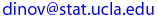# Due Date: Friday, May 09, 2003, turn in after lecture

Correct solutions to any four problems carry full credit, but you must complete problem HW3_5. See the HW submission rules. On the front page include the following header.

• (HW_3_1)  [Sec. 3.1,  #4]  Let X = the number of nonzero digits in a randomly selected zip code. What are the possible values of X? Give three possible outcomes and their associated X values.

• (HW_3_2)  [Sec. 3.2,  #12]  Let X = the number of tires on a randomly selected automobile that are underinflated.
(a) Which of the following three p(x) functions is a legitimate probability mass function for X, and what are the other two not allowed?
 x 0 1 2 3 4 p1(x) 0.3 0.2 0.1 0.05 0.05 p2(x) 0.4 0.1 0.1 0.1 0.3 p3(x) 0.4 0.1 0.2 0.1 0.3

(b) For the legitimate pmf of part (a), compute P(2 ≤ X ≤ 4), P(X ≤ 2), and P(X ≠ 0).
(c) If p(x) = c (5 − x) for x = 0, 1, 2, 3, 4, what is the correct value of c?

• (HW_3_3) [Sec. 3.2,  #13]  A mail-order computer business has six telephone lines. Let X denote the number of lines in use at a specified time. Suppose the pmf of X is as given in the accompanying table. Calculate the probability of each of the following probabilities:  x 0 1 2 3 4 5 6 p(x) 0.1 0.15 0.2 0.25 0.2 0.06 0.04

(a) {at most 3 lines are in use}
(b) {fewer than 3 lines are in use}
(c) {at least 3 lines are in use}
(d) {between 2 and 5 lines, inclusive, are in use}
(e) {between 2 and 4 lines, inclusive, are not in use}
(f) {at least 4 lines are not in use}

• (HW_3_4) [Sec. 3.3,  #28] The pmf for X = the number of major defects on a randomly selected applicance of a certain type is given in the table below. Compute the following:
 x 0 1 2 3 4 p(x) 0.08 0.15 0.45 0.27 0.05
(a) E(X), the expected value of the R.V. X.
(b) V(X), the variance of X.
(c) SD(X), the standard deviation of X.
(d) V(X), using the shortcut formula, V(X)  =  E(X2 ) − µ2. Should equal the answer in part (b).

• (HW_3_5) [Sec. 3.3,  #31] An appliance dealer sells three different models of upright freezers having 13.5 ft3, 15.9 ft3, and 19.1 ft3 (cubic feet) of storage space, respectively. Let X = the amount of storage space purchased by the next customer to buy a freezer. Suppose that X has pmf.
 x 13.5 15.9 19.1 p(x) 0.2 0.5 0.3

(a) Compute E(X), E(X2), E(X3), and V(X).
(b) If the price of a freezer having capacity X ft3 is 25X − 8.5, what is the expected price paid by the next customer to buy a freezer?
(c) What is the variance of the price 25X − 8.5 paid by the next customer? Interpret this value!
(d) Suppose that although the rated capacity of a freezer is X, the actual capacity is h(X) = X − 0.01X2, slightly under the advertised space. What is the expected actual capacity of the freezer purchased by the next customer?

Last modified on by.

Ivo D. Dinov, Ph.D., Departments of Statistics and Neurology, UCLA School of Medicine